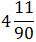# expressed in the form of p/q equals:

1.  4/11

2.  4/13

3.  35/90

4.  35/99

4

4/11

Explanation :
No Explanation available for this question

# The least among the following is:

1.  0.2

2.  1 + 0.2

3.

4.  (0.2)2

4

(0.2)2

Explanation :
No Explanation available for this question

# The correct expression ofin the fractional form is:

1.  646/99

2.  64640/1000

3.  640/100

4.  640/99

4

640/99

Explanation :
No Explanation available for this question

# The value of is:

1.  57/10

2.  57/99

3.  26/45

4.  52/9

4

26/45

Explanation :
No Explanation available for this question

# Mr. Das is M.Com and his date of birth is 30.6.1961

1.  Mark answer (a) if the candidate can be selected

2.  mark answer (b) if the candidate is to be referred to the Director- Finance

3.  mark answer (c) if the candidate can be called for group discussion

4.  mark answer (d) if the candidates can be called for interview

5.  mark answer (e) if the candidate cannot be selected

5

mark answer (c) if the candidate can be called for group discussion

Explanation :
No Explanation available for this question

# Lef F = When F is written as a fraction in lowest terms, the denominator exceeds the numerator by:

1.  13

2.  14

3.  29

4.  87

4

13

Explanation :
No Explanation available for this question

# Mrs. Krishnamurthy is a qualified CA and was 25 years old on 31.7.1987

1.  Mark answer (a) if the candidate can be selected;

2.  mark answer (b) if the candidate is to be referred to the Director- Finance;

3.  mark answer (c) if the candidate can be called for group discussion;

4.  mark answer (d) if the candidates can be called for interview;

5.  mark answer (e) if the candidate cannot be selected

5

mark answer (b) if the candidate is to be referred to the Director- Finance;

Explanation :
No Explanation available for this question

# Mr. Kant, a post graduate with commerce obtained 70% marks in group discussion and secured 20 marks in interview. He was 35 years as on 31.7.1996

1.  Mark answer (a) if the candidate can be selected;

2.  mark answer (b) if the candidate is to be referred to the Director- Finance;

3.  mark answer (c) if the candidate can be called for group discussion;

4.  mark answer (d) if the candidates can be called for interview;

5.  mark answer (e) if the candidate cannot be selected

5

Mark answer (a) if the candidate can be selected;

Explanation :
No Explanation available for this question

# The value ofis:

1.

2.

3.  371/900

4.  None of these

4Explanation :
No Explanation available for this question

# Mrs. Desai is a post-graduate in economics and her date of birth is 3.4.1965

1.  Mark answer (a) if the candidate can be selected;

2.  mark answer (b) if the candidate is to be referred to the Director- Finance;

3.  mark answer (c) if the candidate can be called for group discussion;

4.  mark answer (d) if the candidates can be called for interview;

5.  mark answer (e) if the candidate cannot be selected

5